# Trees - B-Tree Notes | Study Programming and Data Structures - Computer Science Engineering (CSE)

## Computer Science Engineering (CSE): Trees - B-Tree Notes | Study Programming and Data Structures - Computer Science Engineering (CSE)

The document Trees - B-Tree Notes | Study Programming and Data Structures - Computer Science Engineering (CSE) is a part of the Computer Science Engineering (CSE) Course Programming and Data Structures.
All you need of Computer Science Engineering (CSE) at this link: Computer Science Engineering (CSE)

Introduction

B-Tree is a self-balancing search tree. In most of the other self-balancing search trees (like AVL and Red-Black Trees), it is assumed that everything is in main memory. To understand the use of B-Trees, we must think of the huge amount of data that cannot fit in main memory. When the number of keys is high, the data is read from disk in the form of blocks. Disk access time is very high compared to the main memory access time. The main idea of using B-Trees is to reduce the number of disk accesses. Most of the tree operations (search, insert, delete, max, min, ..etc ) require O(h) disk accesses where h is the height of the tree. B-tree is a fat tree. The height of B-Trees is kept low by putting maximum possible keys in a B-Tree node. Generally, the B-Tree node size is kept equal to the disk block size. Since the height of the B-tree is low so total disk accesses for most of the operations are reduced significantly compared to balanced Binary Search Trees like AVL Tree, Red-Black Tree, ..etc.

Time Complexity of B-Tree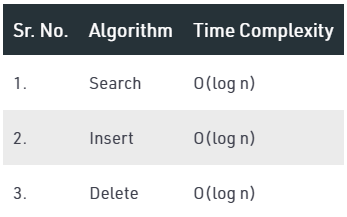“n” is the total number of elements in the B-tree.

Properties of B-Tree

1. All leaves are at the same level.
2. A B-Tree is defined by the term minimum degree ‘t’. The value of t depends upon disk block size.
3. Every node except root must contain at least (ceiling)([t-1]/2) keys. The root may contain minimum 1 key.
4. All nodes (including root) may contain at most t – 1 keys.
5. Number of children of a node is equal to the number of keys in it plus 1.
6. All keys of a node are sorted in increasing order. The child between two keys k1 and k2 contains all keys in the range from k1 and k2.
7. B-Tree grows and shrinks from the root which is unlike Binary Search Tree. Binary Search Trees grow downward and also shrink from downward.
8. Like other balanced Binary Search Trees, time complexity to search, insert and delete is O(log n).

Following is an example of B-Tree of minimum order 5. Note that in practical B-Trees, the value of the minimum order is much more than 5.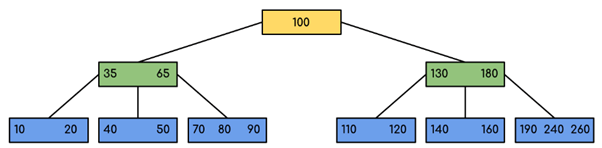We can see in the above diagram that all the leaf nodes are at the same level and all non-leaf have no empty sub-tree and have keys one less than the number of their children.

Interesting Facts

1. The minimum height of the B-Tree that can exist with n number of nodes and m is the maximum number of children of a node can have is: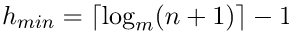2. The maximum height of the B-Tree that can exist with n number of nodes and d is the minimum number of children that a non-root node can have is: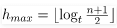and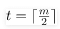Traversal in B-Tree: Traversal is also similar to Inorder traversal of Binary Tree. We start from the leftmost child, recursively print the leftmost child, then repeat the same process for remaining children and keys. In the end, recursively print the rightmost child.

Search Operation in B-Tree: Search is similar to the search in Binary Search Tree. Let the key to be searched be k. We start from the root and recursively traverse down. For every visited non-leaf node, if the node has the key, we simply return the node. Otherwise, we recur down to the appropriate child (The child which is just before the first greater key) of the node. If we reach a leaf node and don’t find k in the leaf node, we return NULL.

Logic:  Searching a B-Tree is similar to searching a binary tree. The algorithm is similar and goes with recursion. At each level, the search is optimised as if the key value is not present in the range of parent then the key is present in another branch. As these values limit the search they are also known as limiting value or separation value. If we reach a leaf node and don’t find the desired key then it will display NULL.

Example: Searching 120 in the given B-Tree.Solution: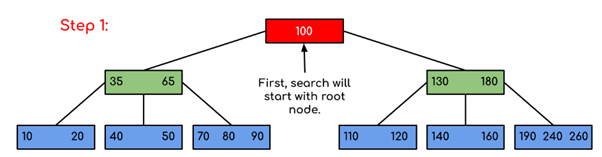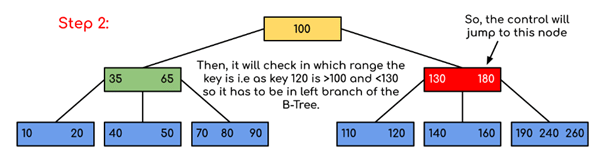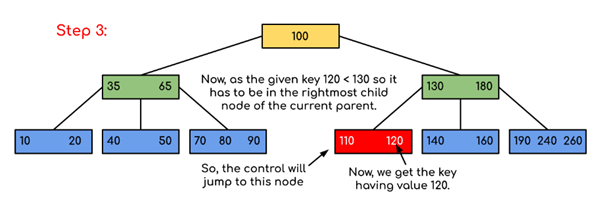In this example, we can see that our search was reduced by just limiting the chances where the key containing the value could be present. Similarly if within the above example we’ve to look for 180, then the control will stop at step 2 because the program will find that the key 180 is present within the current node. And similarly, if it’s to seek out 90 then as 90 < 100 so it’ll go to the left subtree automatically and therefore the control flow will go similarly as shown within the above example.

• C++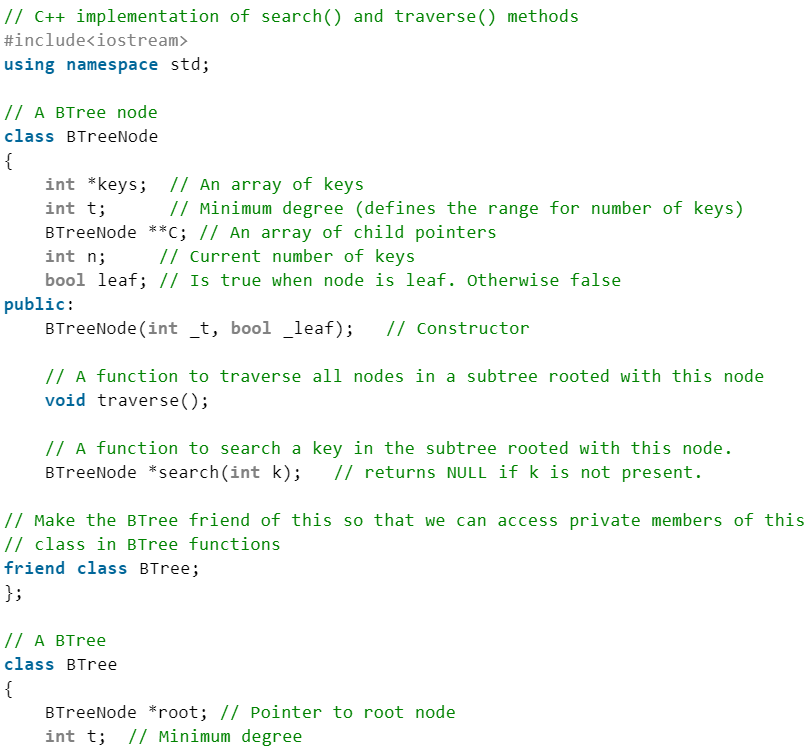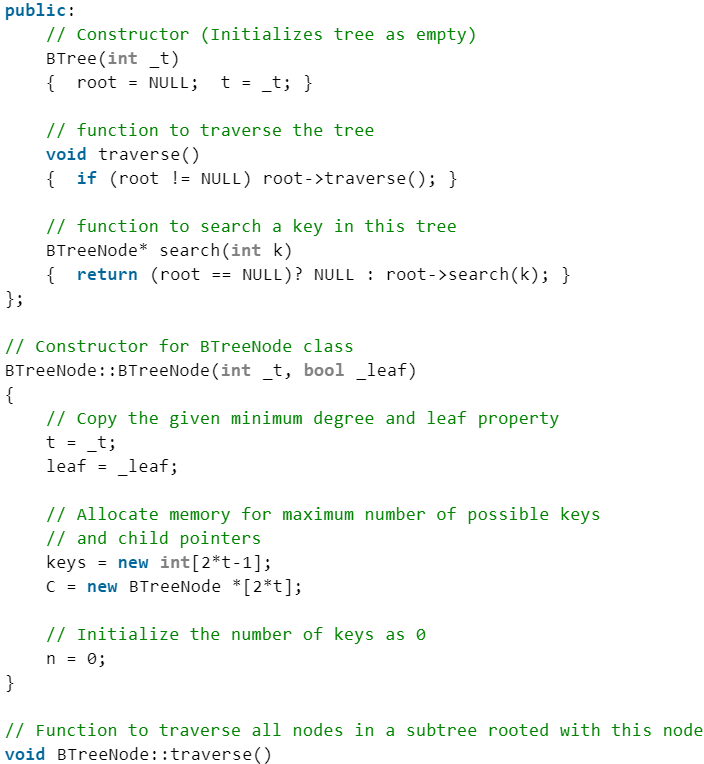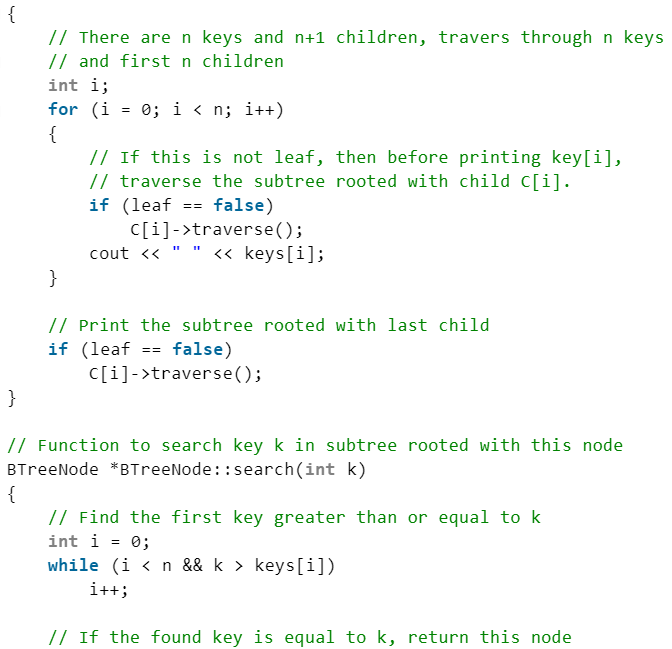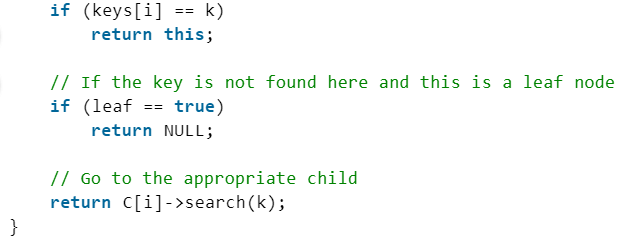• Java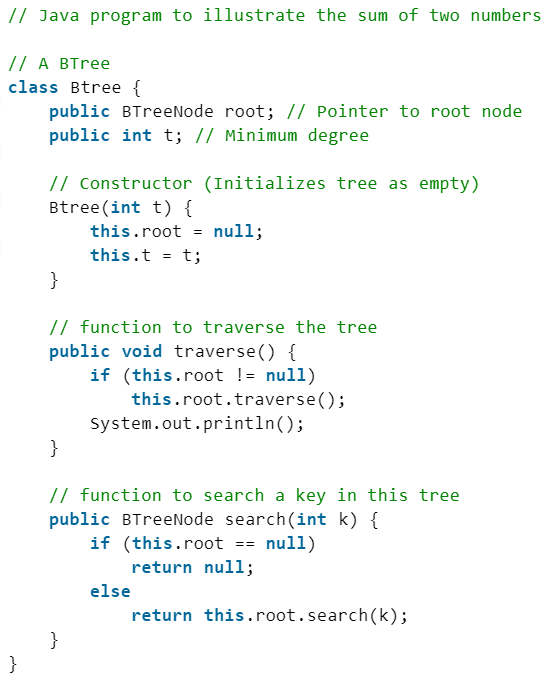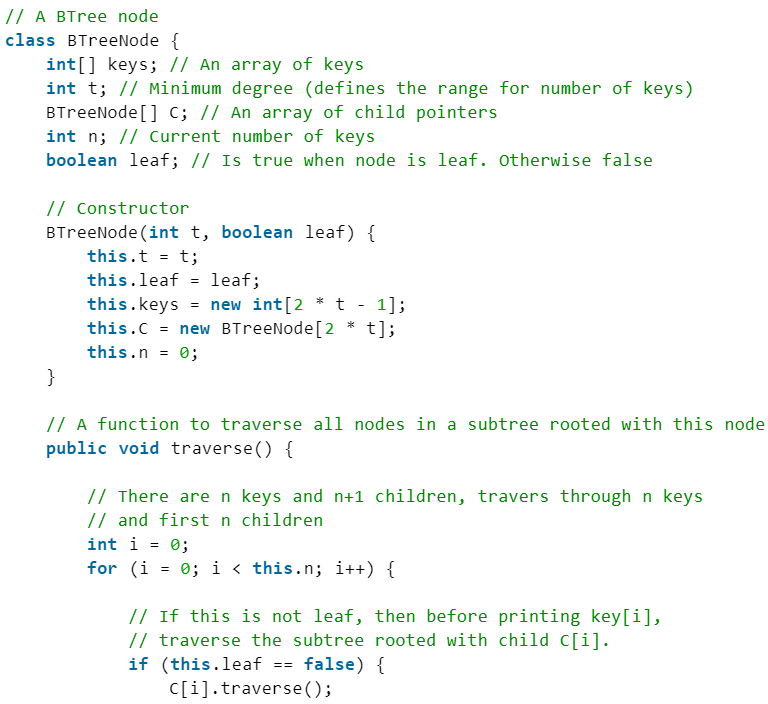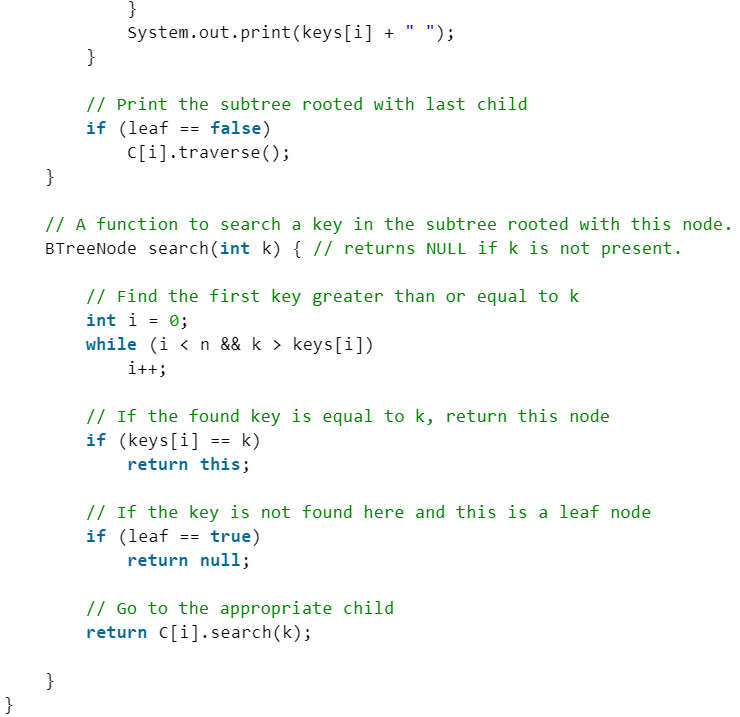• C#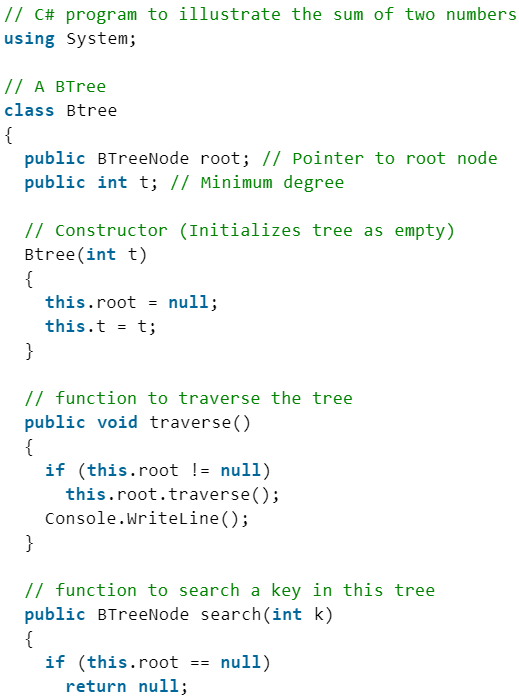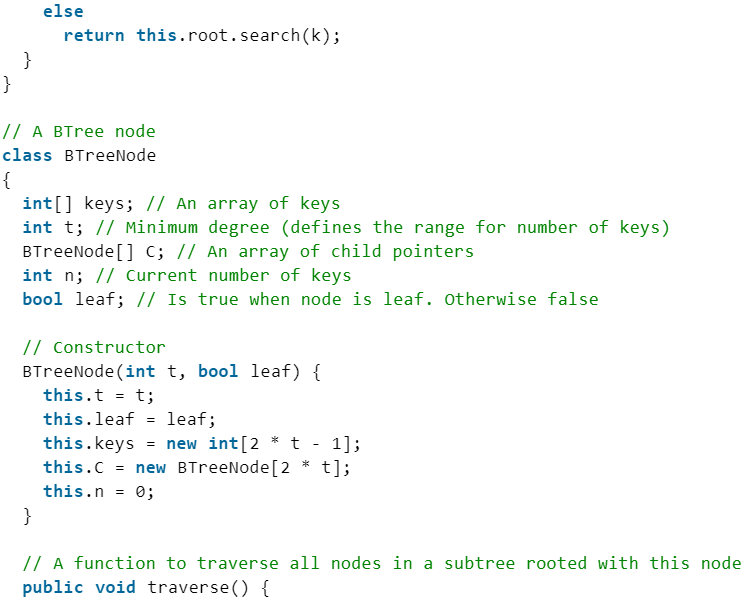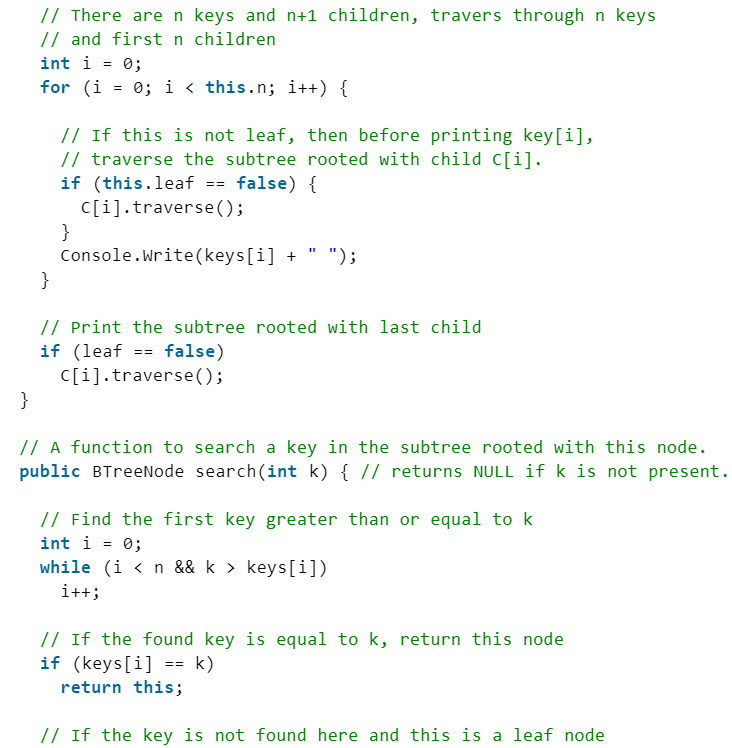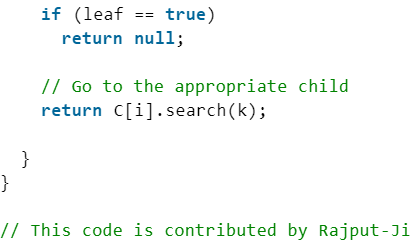The document Trees - B-Tree Notes | Study Programming and Data Structures - Computer Science Engineering (CSE) is a part of the Computer Science Engineering (CSE) Course Programming and Data Structures.
All you need of Computer Science Engineering (CSE) at this link: Computer Science Engineering (CSE)Use Code STAYHOME200 and get INR 200 additional OFF

## Programming and Data Structures

56 docs|17 tests

Track your progress, build streaks, highlight & save important lessons and more!

,

,

,

,

,

,

,

,

,

,

,

,

,

,

,

,

,

,

,

,

,

;